##### Econometrics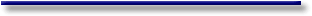# Online Econometrics Textbook - Regression Extensions - Assumption Violations of Linear Regression - Heteroskedasticity in Linear Regression

#### III.I.1 Heteroskedasticity in Linear Regression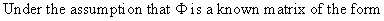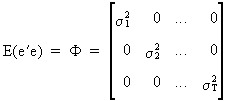(III.I.1-1)

we can use a two step procedure to solve the problem of heteroskedasticity as follows: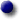divide each observation by the S.D. of the error term for that observationapply LS to the transformed observations

This procedure is called the Weighted Least Squares (WLS).

Of course there exist a lot of alternative transformations. One of the most popular transformations is the Neperian logarithm, since it gives more weight to small-valued observations and less weight to large ones. The transformation of time series according to the logarithmic and related transformations is (in econometrics) mostly assumed to be theory-related.

Another method is specially designed to solve the problem of multiplicative heteroskedasticity.

Suppose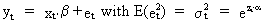(III.I.1-2)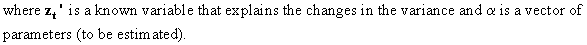From (III.I.1-2) we find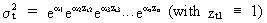(III.I.1-3)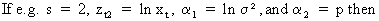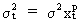(III.I.1-4)

The only question remaining is "How to estimate alpha?".

We may rewrite (III.I.1-2) by taking logarithms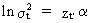(III.I.1-5)

and since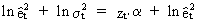(III.I.1-6)

it is obvious that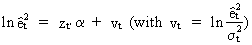(III.I.1-7)

Now we put all t elements from (III.I.1-7) in matrices and obtain estimates of alpha for the model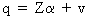(III.I.1-8)

by solving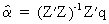(III.I.1-9)

Once the alpha parameter vector has been computed this information can be used in the following Estimated Generalized Least Squares estimator (EGLS)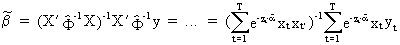(III.I.1-10)

Consider the following multiple regression equation (to be used in subsequent illustrations):

Estimation with OLS:

Endogenous variable = ship.dba

Variable Parameter S.E. t-stat

const(-0),1.,0,0 +294.5647227 247.2103565 +1.19 employ(-0),1.,0,0 +34.36683831 5.160885579 +6.66 expend(-0),1.,0,0 +9.572870953 2.108664727 +4.54

2-tail-t at 95 percent = 2.042
1-tail-t at 95 percent = 1.697
R-squared of stationary series = 0.9193525203 Durbin-Watson = 2.472087712
Variance of regression = 1051039.479
Standard Error of regression = 1025.202165
Sum of Squared Residuals = 37837421.25
Degrees of freedom = 36

Correlation matrix of parameters:

+1.00 -0.42 +0.03
-0.42 +1.00 -0.85
+0.03 -0.85 +1.00

Detection of heteroskedasticity can be achieved by many different tests. If we assume a linear statistical model of the form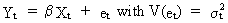(III.I.1-11)

then a test for heteroskedasticity, according to Glejser, can be obtained by testing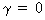in one of the following models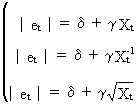(III.I.1-12)

(and many others...).

Warning: this test should only be used if the endogenous variable is NOT used as lagged exogenous variable. Furthermore the Gleisjer tests assume ADDITIVE heteroskedasticity. All OLS assumptions should be satisfied.

Below you ll find an example of how Gleisjer tests can be applied to test for heteroskedasticity (this test is applied to our example-equation):

Gleisjer tests:

Estimation with OLS:

Endogenous variable = abs(e)

Variable Parameter S.E. t-stat

const(-0),1.,0,0 +186.2884948 148.8382962 +1.25
employ(-0),1.,0,0 +7.009636535 1.65355995 +4.24

2-tail-t at 95 percent = 2.042
1-tail-t at 95 percent = 1.697

R-squared of stationary series = 0.3392877362 Durbin-Watson = 2.107710811
Degrees of freedom = 37
Variance of regression = 381232.1935
Standard Error of regression = 617.4400323
Sum of Squared Residuals = 14105591.16

Correlation matrix of parameters:

+1.00 -0.75
-0.75 +1.00

T-STAT of b in abs(e) = a + b X = 4.239118477

Estimation with OLS:

Endogenous variable = abs(e)

Variable Parameter S.E. t-stat

const(-0),1.,0,0 +247.1919656 131.7411655 +1.88
expend(-0),1.,0,0 +3.009214899 0.658345735 +4.57

2-tail-t at 95 percent = 2.042
1-tail-t at 95 percent = 1.697

R-squared of stationary series = 0.3613379003 Durbin-Watson = 1.709541158
Degrees of freedom = 37
Variance of regression = 361985.459
Standard Error of regression = 601.6522741
Sum of Squared Residuals = 13393461.98

Correlation matrix of parameters:

+1.00 -0.68
-0.68 +1.00

T-STAT of b in abs(e) = a + b X = 4.570873234

Estimation with OLS:

Endogenous variable = abs(e)

Variable Parameter S.E. t-stat

const(-0),1.,0,0 +1453.542646 204.7255451 +7.1
employ(-0),1.,0,0 -34269.28262 7753.841264 -4.42

2-tail-t at 95 percent = 2.042
1-tail-t at 95 percent = 1.697

R-squared of stationary series = 0.3458859921 Durbin-Watson = 1.762971931
Degrees of freedom = 37
Variance of regression = 370690.754
Standard Error of regression = 608.8437845
Sum of Squared Residuals = 13715557.9

Correlation matrix of parameters:

+1.00 -0.88
-0.88 +1.00

T-STAT of b in abs(e) = a + b 1/X = -4.419652331

Estimation with OLS:

Endogenous variable = abs(e)

Variable Parameter S.E. t-stat

const(-0),1.,0,0 +1296.342082 182.4520168 +7.11
expend(-0),1.,0,0 -42579.02335 10204.88361 -4.17

2-tail-t at 95 percent = 2.042
1-tail-t at 95 percent = 1.697

R-squared of stationary series = 0.3203453806 Durbin-Watson = 1.553508102
Degrees of freedom = 37
Variance of regression = 385163.4666
Standard Error of regression = 620.6153935
Sum of Squared Residuals = 14251048.26

Correlation matrix of parameters:

+1.00 -0.84
-0.84 +1.00

T-STAT of b in abs(e) = a + b 1/X = -4.172416362

Estimation with OLS:

Endogenous variable = abs(e)

Variable Parameter S.E. t-stat

const(-0),1.,0,0 -566.1796621 258.3961199 -2.19
employ(-0),1.,0,0 +159.8350094 31.50187299 +5.07

2-tail-t at 95 percent = 2.042
1-tail-t at 95 percent = 1.697

R-squared of stationary series = 0.4106281997 Durbin-Watson = 2.169617364
Degrees of freedom = 37
Variance of regression = 333999.7394
Standard Error of regression = 577.9271056
Sum of Squared Residuals = 12357990.36

Correlation matrix of parameters:

+1.00 -0.93
-0.93 +1.00

T-STAT of b in abs(e) = a + b sqrt(X) = 5.073825594

Estimation with OLS:

Endogenous variable = abs(e)

Variable Parameter S.E. t-stat

const(-0),1.,0,0 -318.6307381 205.1846763 -1.55
expend(-0),1.,0,0 +93.21309421 17.56301183 +5.31

2-tail-t at 95 percent = 2.042
1-tail-t at 95 percent = 1.697

R-squared of stationary series = 0.4325531446 Durbin-Watson = 1.866343044
Degrees of freedom = 37
Variance of regression = 321574.7705
Standard Error of regression = 567.0756303
Sum of Squared Residuals = 11898266.51

Correlation matrix of parameters:

+1.00 -0.90
-0.90 +1.00

T-STAT of b in abs(e) = a + b sqrt(X) = 5.30735247

Estimation with OLS:

Endogenous variable = abs(e)

Variable Parameter S.E. t-stat

const(-0),1.,0,0 +186.2884948 148.8382962 +1.25
employ(-0),1.,0,0 +7.009636535 1.65355995 +4.24

2-tail-t at 95 percent = 2.042
1-tail-t at 95 percent = 1.697

R-squared of stationary series = 0.3272823951 Durbin-Watson = 2.107710811
Degrees of freedom = 37
Variance of regression = 381232.1935
Standard Error of regression = 617.4400323
Sum of Squared Residuals = 14105591.16

Correlation matrix of parameters:

+1.00 -0.75
-0.75 +1.00

T-STAT of b in abs(e) = a + b abs(X) = 4.239118477

Estimation with OLS:

Endogenous variable = abs(e)

Variable Parameter S.E. t-stat

const(-0),1.,0,0 +247.1919656 131.7411655 +1.88
expend(-0),1.,0,0 +3.009214899 0.658345735 +4.57

2-tail-t at 95 percent = 2.042
1-tail-t at 95 percent = 1.697

R-squared of stationary series = 0.3612449444 Durbin-Watson = 1.709541158
Degrees of freedom = 37
Variance of regression = 361985.459
Standard Error of regression = 601.6522741
Sum of Squared Residuals = 13393461.98

Correlation matrix of parameters:

+1.00 -0.68
-0.68 +1.00

T-STAT of b in abs(e) = a + b abs(X) = 4.570873234

Estimation with OLS:

Endogenous variable = abs(e)

Variable Parameter S.E. t-stat

const(-0),1.,0,0 +534.6889586 121.5421391 +4.4
employ(-0),1.,0,0 +1.52089658e-002 6.025380217e-003 +2.52

2-tail-t at 95 percent = 2.042
1-tail-t at 95 percent = 1.697

R-squared of stationary series = 0.1473776172 Durbin-Watson = 1.732573146
Degrees of freedom = 37
Variance of regression = 483185.0673
Standard Error of regression = 695.1151468
Sum of Squared Residuals = 17877847.49

Correlation matrix of parameters:

+1.00 -0.40
-0.40 +1.00

T-STAT of b in abs(e) = a + b X*X = 2.524150387

Estimation with OLS:

Endogenous variable = abs(e)

Variable Parameter S.E. t-stat

const(-0),1.,0,0 +509.6383822 120.1542444 +4.24
expend(-0),1.,0,0 +3.702769252e-003 1.275457967e-003 +2.9

2-tail-t at 95 percent = 2.042
1-tail-t at 95 percent = 1.697

R-squared of stationary series = 0.1859772184 Durbin-Watson = 1.408225521
Degrees of freedom = 37
Variance of regression = 461310.494
Standard Error of regression = 679.1984202
Sum of Squared Residuals = 17068488.28

Correlation matrix of parameters:

+1.00 -0.43
-0.43 +1.00

T-STAT of b in abs(e) = a + b X*X = 2.903089987

Another popular test is the so-called likelihood ratio test for heteroskedasticity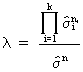(III.I.1-13)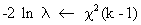(III.I.1-14)

which can be used for testing statistical significance.

The Goldfeld-Quandt test for heteroskedasticity uses test-equations for each exogenous variable (except the constant term). This test is widely applicable, and fairly unproblematic w.r.t. its properties. The Goldfeld-Quandt test uses two regressions of the endogenous variable on each variable separately; the first regression is based on LOW values of the exogenous variable, the second regression is based on HIGH values of the exogenous variable. Note that a pre-specified number of values in-between LOW and HIGH values are NOT used in these regressions.

Below you ll find an example of how the Goldfeld-Quandt test can be applied to test for heteroskedasticity (this test is applied to our example-equation):

Goldfeld-Quandt Test

The Goldfeld-Quandt test will be based on two regressions of (T - 13)/2 observations. The first regression on low values of Xi, the second on high values of Xi.

Estimation with OLS:

Endogenous variable = ship.dba

Variable Parameter S.E. t-stat

const(-0),1.,0,0 +1795.714286 130.620347 +13.7

2-tail-t at 95 percent = 2.16
1-tail-t at 95 percent = 1.771

R-squared of stationary series = 0.2605089242 Durbin-Watson = 2.718602812
Degrees of freedom = 13
Variance of regression = 238863.4505
Standard Error of regression = 488.7365861
Sum of Squared Residuals = 3105224.857

Estimation with OLS:

Endogenous variable = ship.dba

Variable Parameter S.E. t-stat

const(-0),1.,0,0 +6985.071429 1153.776244 +6.05

2-tail-t at 95 percent = 2.16
1-tail-t at 95 percent = 1.771

R-squared of stationary series = 1.268073674e-003 Durbin-Watson = 1.01313106
Degrees of freedom = 13
Variance of regression = 18636794.69
Standard Error of regression = 4317.035405
Sum of Squared Residuals = 242278330.9

Goldfeld-Quandt test for exogenous variable nr. 1 = 78.02279773
DF of numerator = DF of denominator.
Approximate F critical value (95%) (df = {13,13}) = 2.4

Estimation with OLS:

Endogenous variable = ship.dba

Variable Parameter S.E. t-stat

employ(-0),1.,0,0 +62.53440159 2.987781852 +20.9

2-tail-t at 95 percent = 2.16
1-tail-t at 95 percent = 1.771

R-squared of stationary series = 0.9195408482 Durbin-Watson = 1.804461765
Degrees of freedom = 13
Variance of regression = 98605.87903
Standard Error of regression = 314.0157305
Sum of Squared Residuals = 1281876.427

Estimation with OLS:

Endogenous variable = ship.dba

Variable Parameter S.E. t-stat

employ(-0),1.,0,0 +56.49942486 3.939457673 +14.3

2-tail-t at 95 percent = 2.16
1-tail-t at 95 percent = 1.771

R-squared of stationary series = 1.003004769 Durbin-Watson = 1.178141189
Degrees of freedom = 13
Variance of regression = 4357873.512
Standard Error of regression = 2087.552038
Sum of Squared Residuals = 56652355.66

Goldfeld-Quandt test for exogenous variable nr. 2 = 44.194865
DF of numerator = DF of denominator.
Approximate F critical value (95%) (df = {13,13}) = 2.4

Estimation with OLS:

Endogenous variable = ship.dba

Variable Parameter S.E. t-stat

expend(-0),1.,0,0 +43.94765568 3.306817926 +13.3

2-tail-t at 95 percent = 2.16
1-tail-t at 95 percent = 1.771

R-squared of stationary series = 0.8503625979 Durbin-Watson = 2.958320856
Degrees of freedom = 13
Variance of regression = 254447.5573
Standard Error of regression = 504.4279505
Sum of Squared Residuals = 3307818.245

Estimation with OLS:

Endogenous variable = ship.dba

Variable Parameter S.E. t-stat

expend(-0),1.,0,0 +24.00368738 1.992895783 +12.

2-tail-t at 95 percent = 2.16
1-tail-t at 95 percent = 1.771

R-squared of stationary series = 0.8703061351 Durbin-Watson = 2.720031672
Degrees of freedom = 13
Variance of regression = 5853973.458
Standard Error of regression = 2419.498596
Sum of Squared Residuals = 76101654.96

Goldfeld-Quandt test for exogenous variable nr. 3 = 23.00660113
DF of numerator = DF of denominator.
Approximate F critical value (95%) (df = {13,13}) = 2.4

The Park tests for heteroskedasticity uses a test-equation for each exogenous variable: the logarithms of squared residuals are explained by the logarithm of the absolute values of the exogenous variable.

Warning: this test should only be used if the endogenous variable is NOT used as lagged exogenous variable. Furthermore the Park tests assume MULTIPLICATIVE heteroskedasticity. All OLS assumptions should be satisfied.

Below you will find an example of how the Park test can be applied to test for heteroskedasticity (this test is applied to our example-equation):

T-STAT values of b in Simple Regression:

Estimation with OLS:

Endogenous variable = ln(e*e)

Variable Parameter S.E. t-stat

const(-0),1.,0,0 +2.673616498 2.32005065 +1.15 employ(-0),1.,0,0 +2.255741073 0.5790504391 +3.9

2-tail-t at 95 percent = 2.042
1-tail-t at 95 percent = 1.697

T-STAT of b in ln(e*e) = a + b ln abs(X) = 3.8955865

Estimation with OLS:

Endogenous variable = ln(e*e)

Variable Parameter S.E. t-stat

const(-0),1.,0,0 +3.990749162 2.084773124 +1.91 expend(-0),1.,0,0 +1.688936824 0.4553619992 +3.71

2-tail-t at 95 percent = 2.042
1-tail-t at 95 percent = 1.697

T-STAT of b in ln(e*e) = a + b ln abs(X) = 3.708998175

The Breusch-Pagan test for heteroskedasticity uses a test-equation: the squared residuals divided by the residual variance are explained by all exogenous variables. The test statistic is computed as half the difference between the Total Sum of Squares and the Sum of Squared Residuals, which has a Chi-square distribution.

Warning: this test should only be used if the endogenous variable is NOT used as lagged exogenous variable AND if the number of observations is VERY LARGE. All OLS assumptions should be satisfied, including normality of the error term.

Below you ll find an example of how the Breusch-Pagan test can be applied to test for heteroskedasticity (this test is applied to our example-equation):

Breusch-Pagan test:

Estimation with OLS:

Endogenous variable = ê²/(var(ê))

Variable Parameter S.E. t-stat

const(-0),1.,0,0 -5.59940532e-002 0.3553661 -0.158 employ(-0),1.,0,0 +9.716468615e-004 7.418798335e-003 +0.131 expend(-0),1.,0,0 +6.694376613e-003 3.031215889e-003 +2.21

2-tail-t at 95 percent = 2.042
1-tail-t at 95 percent = 1.697

R-squared of stationary series = 0.9998009501 Durbin-Watson = 2.041789465
Degrees of freedom = 36
Variance of regression = 2.171889371
Standard Error of regression = 1.473733141
Sum of Squared Residuals = 78.18801734

regression: e_hat**2/(var(e_hat)) = a + X b + v
residual variance = 2.171889371
(TSS-SSR)/2 = 39.35189667
Chi-square (95 percent) critical value = 5.99

The Squared Residuals versus Squared Fit test for heteroskedasticity uses a test-equation: the squared residuals are explained by the squared interpolation forecast of the original regression. This test is fairly unproblematic and can be used in almost all cases. The t-statistic of the Squared-Fit-parameter indicates whether heteroskedasticity is present or not.

Below you ll find an example of how the Squared Residuals versus Squared Fit test can be applied to test for heteroskedasticity (this test is applied to our example-equation):

Squared Residuals versus Squared Fit:

Estimation with OLS:

Endogenous variable = SquaredResiduals

Variable Parameter S.E. t-stat

constant(-0),1.,0,0 +592305.4721 312179.7592 +1.9 SquaredFit(-1),1.,0,0 +1.432760191e-002 5.425552377e-003 +2.64

2-tail-t at 95 percent = 2.042
1-tail-t at 95 percent = 1.697

R-squared of stationary series = 0.1585866958 Durbin-Watson = 2.020505492
Degrees of freedom = 37
Variance of regression = 3.002200902e+012
Standard Error of regression = 1732686.037
Sum of Squared Residuals = 1.110814334e+014

Correlation matrix of parameters:

+1.00 -0.46
-0.46 +1.00

regression: e_hat*e_hat = a + y_hat*y_hat*b + v

The ARCH(p) test is used to test for Autoregressive Conditional Heteroskedasticity: the squared residuals are explained by its lagged values (p is the number of lags included in the test-equation). The presence of Conditional Heteroskedasticity is tested by the use of an F-statistic.

Below you ll find an example of how the ARCH(p) test can be applied to test for Conditional Heteroskedasticity (this test is applied to our example-equation):

Arch(p) test by Least Squares:

Estimation with OLS:

Endogenous variable = SquaredResiduals

Variable Parameter S.E. t-stat

constant(-0),1.,0,0 +362168.8873 318289.0843 +1.14
SqResid(-1),1.,0,0 +5.744398869e-002 0.1786811315 +0.321
SqResid(-2),1.,0,0 +0.4790710887 0.1723985422 +2.78
SqResid(-3),1.,0,0 +0.2166609567 0.1908881265 +1.14

2-tail-t at 95 percent = 2.042
1-tail-t at 95 percent = 1.697

R-squared of stationary series = 0.3602748235 Durbin-Watson = 1.823445676
Degrees of freedom = 32
Variance of regression = 2.594323444e+012
Standard Error of regression = 1610690.363
Sum of Squared Residuals = 8.301835022e+013

Correlation matrix of parameters:

+1.00 -0.21 -0.22 -0.11
-0.21 +1.00 -0.25 -0.48
-0.22 -0.25 +1.00 -0.24
-0.11 -0.48 -0.24 +1.00

F-stat = 6.007159936
Critical F value (95%) = 2.84© 2000-2022 All rights reserved. All Photographs (jpg files) are the property of Corel Corporation, Microsoft and their licensors. We acquired a non-transferable license to use these pictures in this website.
The free use of the scientific content in this website is granted for non commercial use only. In any case, the source (url) should always be clearly displayed. Under no circumstances are you allowed to reproduce, copy or redistribute the design, layout, or any content of this website (for commercial use) including any materials contained herein without the express written permission.

Information provided on this web site is provided "AS IS" without warranty of any kind, either express or implied, including, without limitation, warranties of merchantability, fitness for a particular purpose, and noninfringement. We use reasonable efforts to include accurate and timely information and periodically updates the information without notice. However, we make no warranties or representations as to the accuracy or completeness of such information, and it assumes no liability or responsibility for errors or omissions in the content of this web site. Your use of this web site is AT YOUR OWN RISK. Under no circumstances and under no legal theory shall we be liable to you or any other person for any direct, indirect, special, incidental, exemplary, or consequential damages arising from your access to, or use of, this web site.

Contributions and Scientific Research: Prof. Dr. E. Borghers, Prof. Dr. P. Wessa
Please, cite this website when used in publications: Xycoon (or Authors), Statistics - Econometrics - Forecasting (Title), Office for Research Development and Education (Publisher), http://www.xycoon.com/ (URL), (access or printout date).